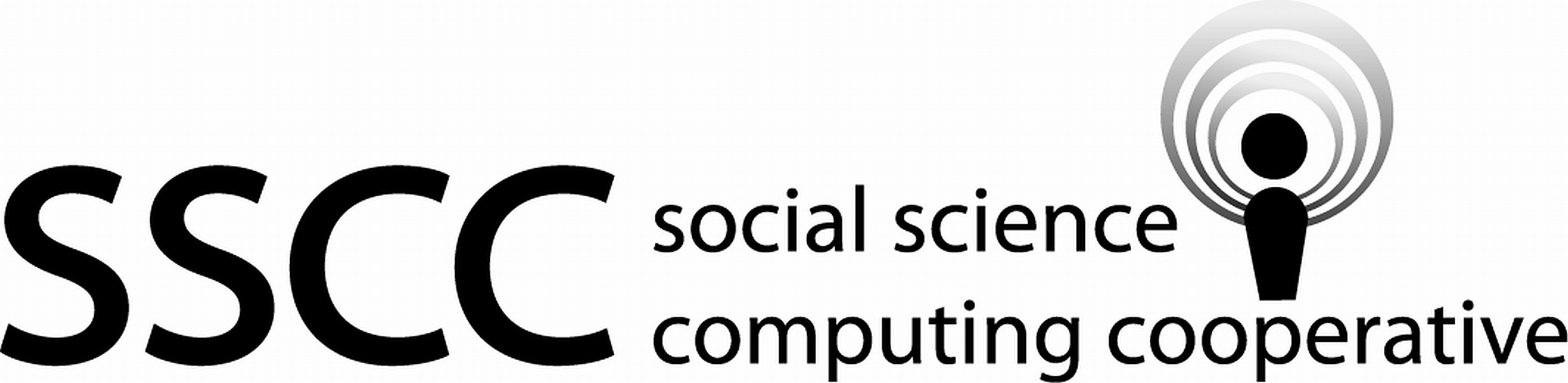Supporting Statistical Analysis for Research

## 3.4 Relationship between more than two variables

These exercises use the `Mroz.csv` data set that was imported in the prior sections of this chapter.

1. Create a scatter plot for `age` against `lwg`. Use color to display women college attendance status.

``````ggplot(mroz, aes(x = age, y = lwg, color = wc)) +
geom_point() +
theme_bw()``````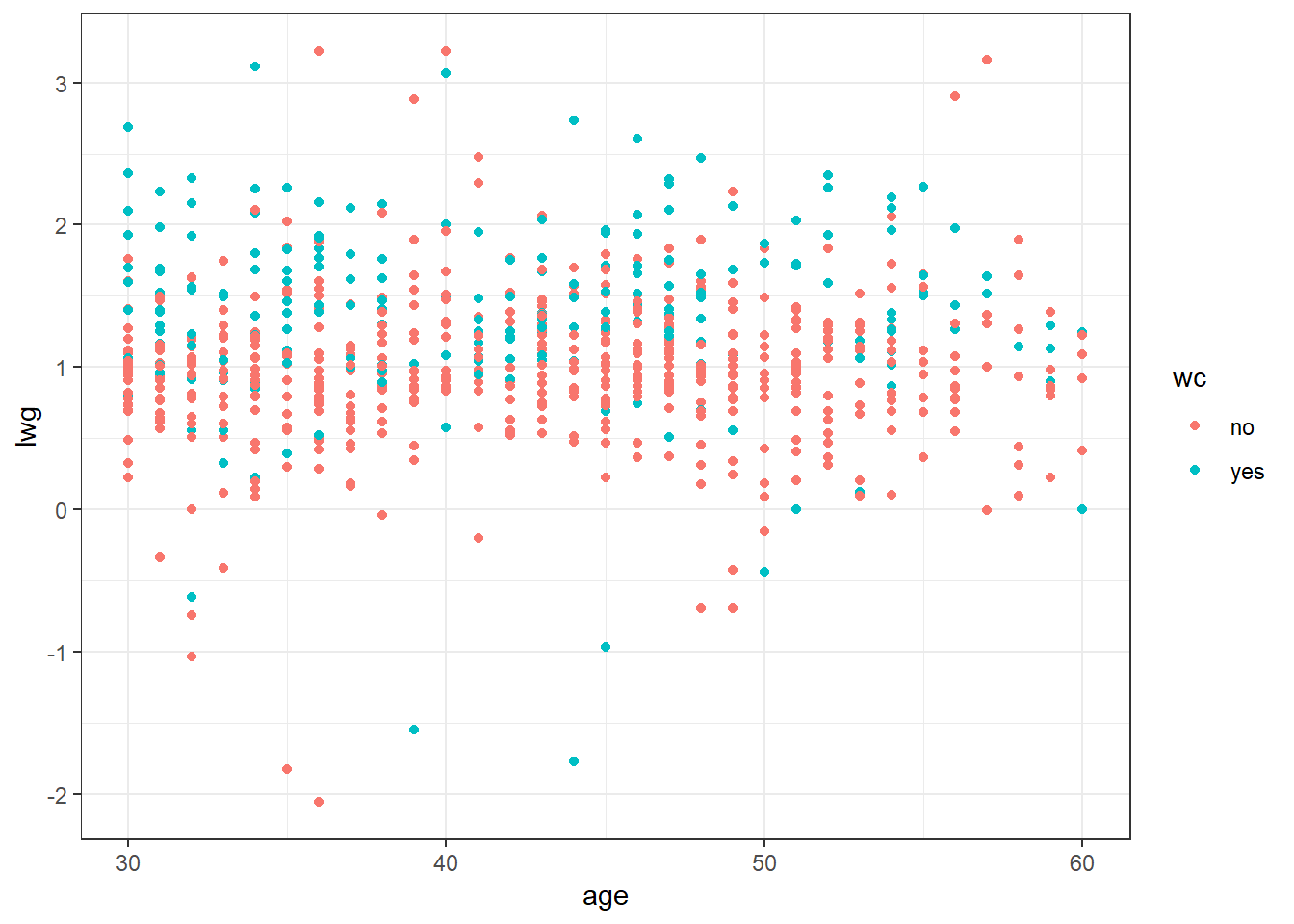2. Facet the prior plot on `hc`.

``````ggplot(mroz, aes(x = age, y = lwg, color = wc)) +
geom_point() +
facet_wrap(~hc) +
theme_bw()``````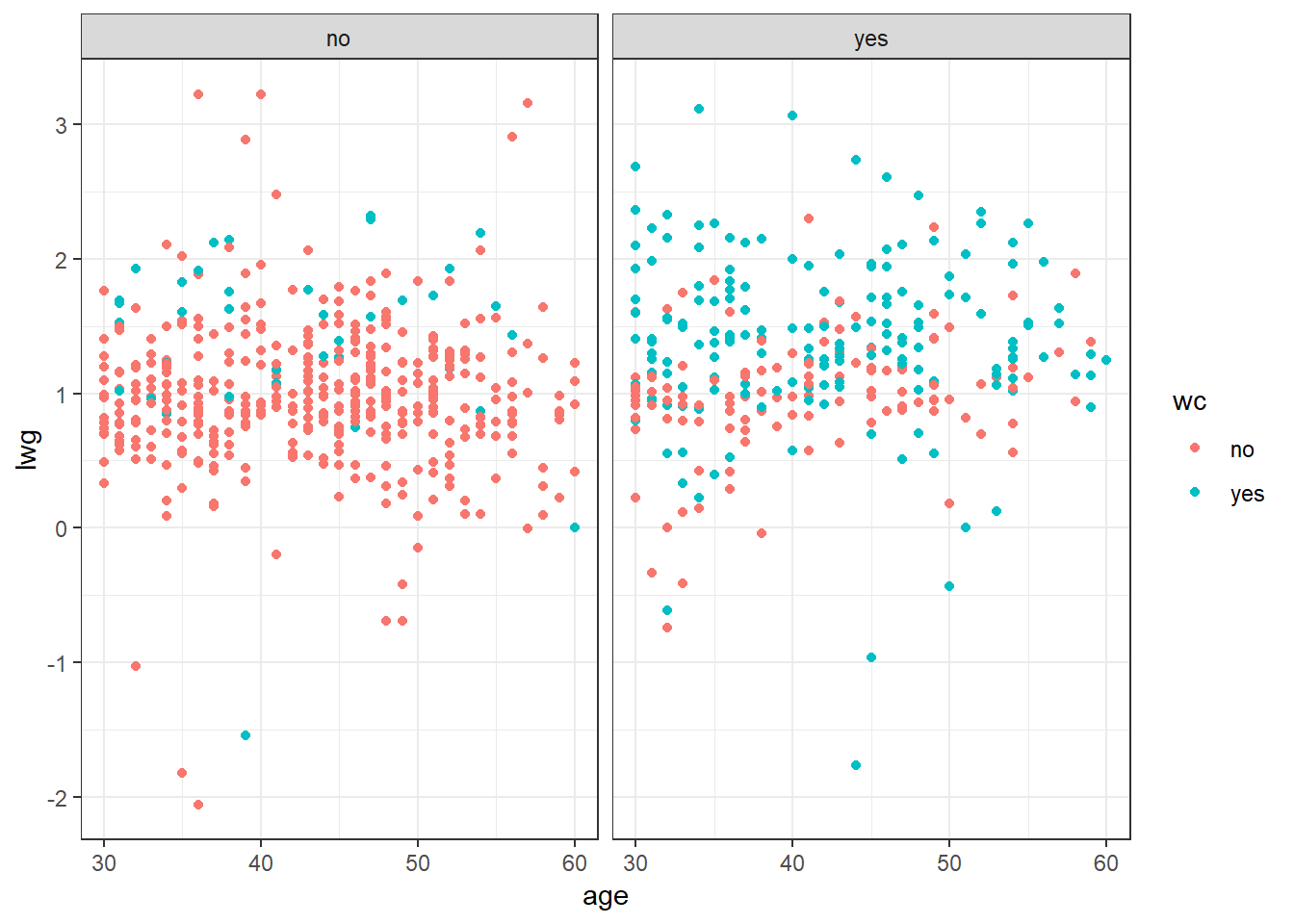3. Add a loess smoothing line `hc`.

``````ggplot(mroz, aes(x = age, y = lwg, color = wc)) +
geom_point() +
geom_smooth(color = "blue") +
facet_wrap(~hc) +
theme_bw()``````
```geom_smooth()` using method = 'loess' and formula 'y ~ x'``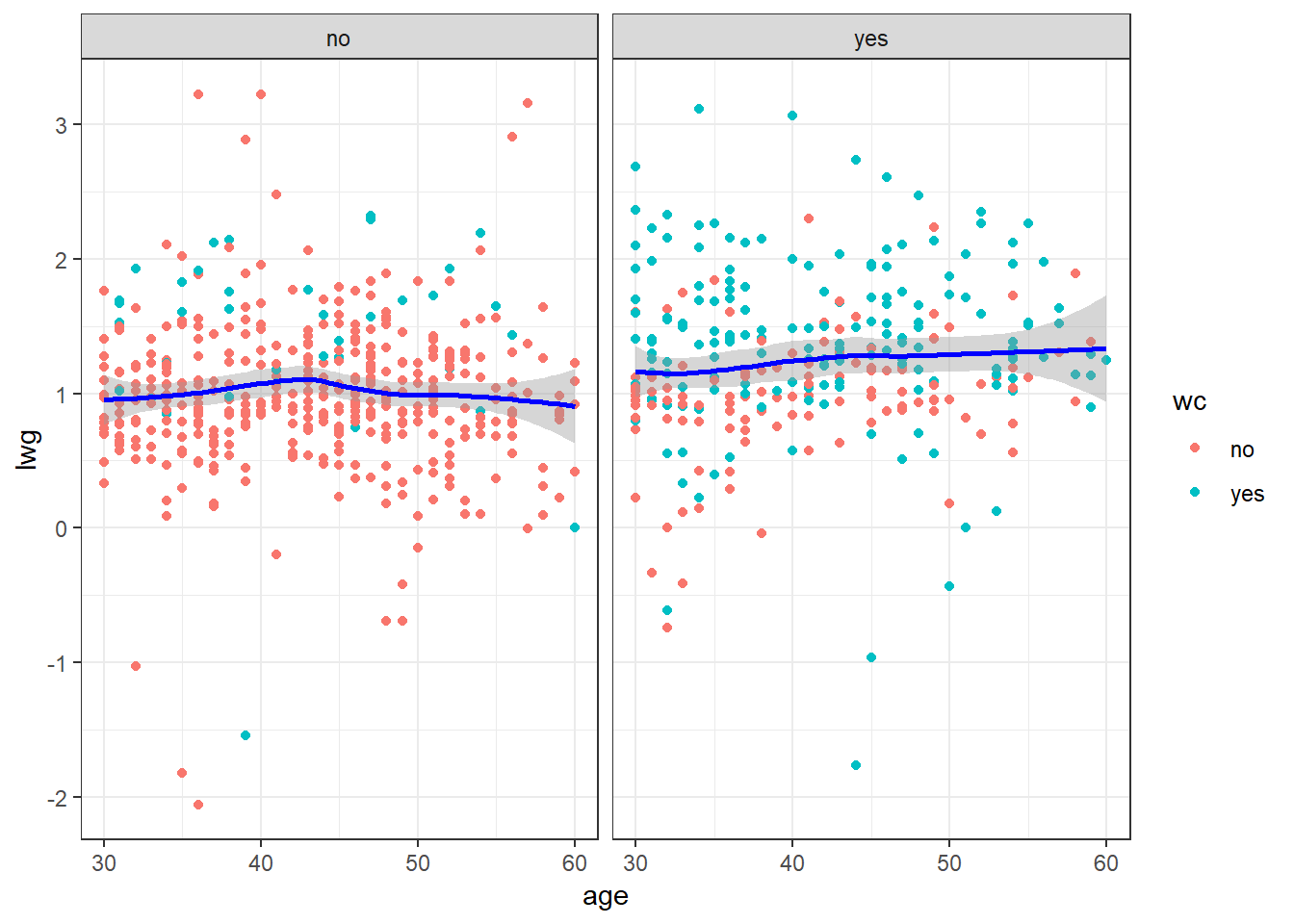4. If the prior plot produces a message or warning, change the code to avoid the warning.

``````ggplot(mroz, aes(x = age, y = lwg, color = wc)) +
geom_point() +
geom_smooth(method = "loess", formula = "y ~ x", color = "blue") +
facet_wrap(~hc) +
theme_bw()``````5. Add a title and provide better axis labels.

``````ggplot(mroz, aes(x = age, y = lwg, color = wc)) +
geom_point() +
geom_smooth(method = "loess", formula = "y ~ x", color = "blue") +
facet_wrap(~hc) +
theme_bw() +
ggtitle("Womens Wages and Ages") +
theme(plot.title = element_text(hjust = 0.5)) +
xlab("Log Womens Wages") +
ylab("Age")``````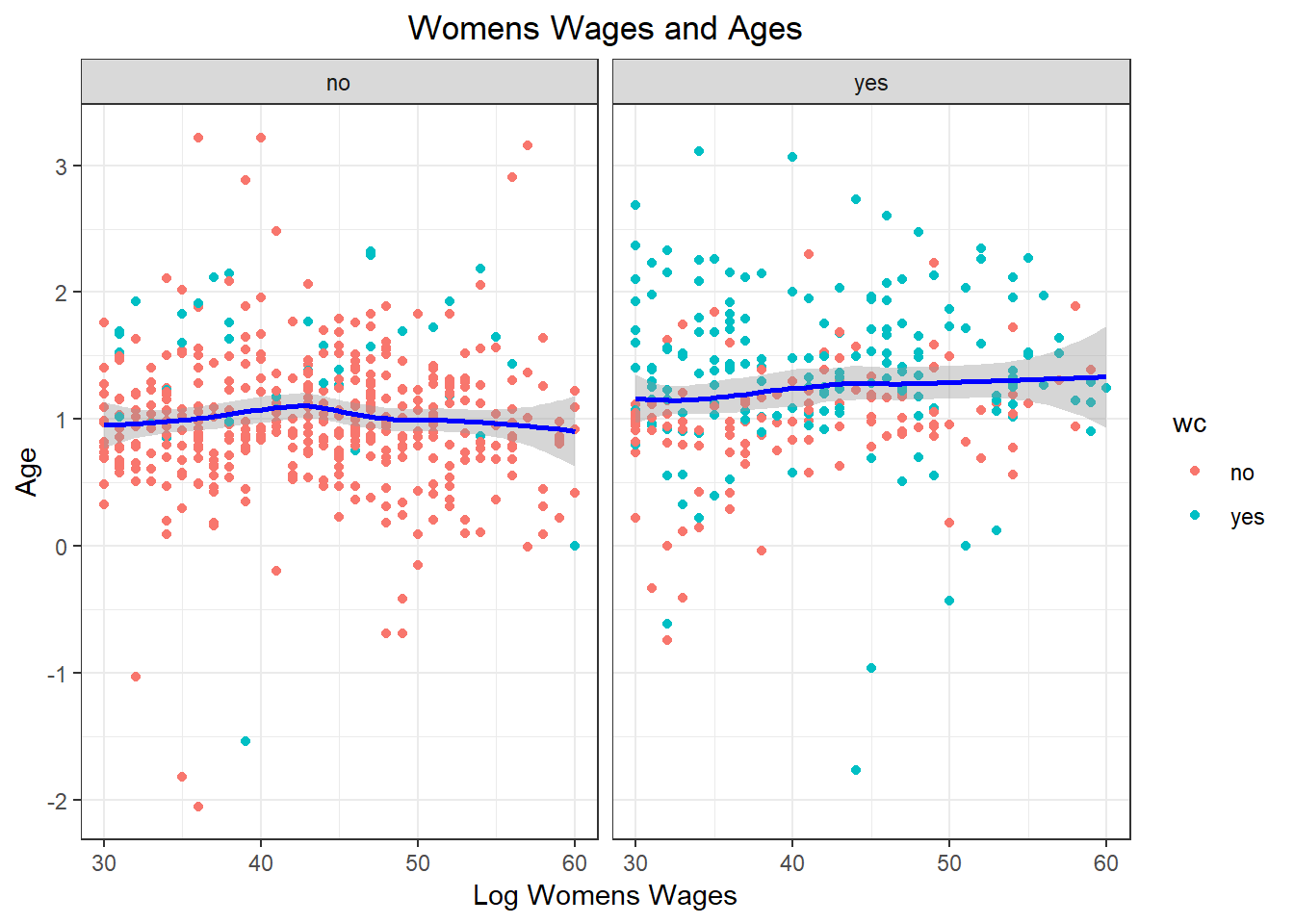6. Create a plot that explores the relationship between at least three variables. Use at least one different value than was used in the prior exercise.